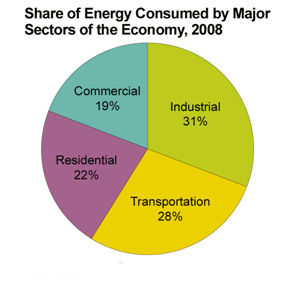FAQ
Home » Blog » FAQ » Sub-transmission network

# Sub-transmission network

Q:
What factors determine current distribution between two 33kV feeders feeding a 33/11kV Substation within a sub-transmission network.

A:
Try the voltage divider rule. Take the R + X of each feeder (resistance and reactance) and find the Z. Remember that square root of R² + X² = Z. Now that you have the Z of each feeder, now find the Z of the two in parallel. To do this we have Z = 1/(1/Z1 +1/Z2). So, if Z1 = 2.16 ohms and Z2 = 1.67 ohms, then our Z of the two in parallel is 0.94 ohms. Now we pass the current of the entire substation between these two feeders. Let’s say that the current is 240 amps. Now we have 240Ax0.94ohms = 226 volts (IxZ=V). And since voltage divided by impedance gives us current (V/Z = I) we now take the voltage drop across the two feeders in parallel and divide each of the feeder impedances to get the separate feeder current. So we get 226V/2.16ohms = 105 amps (feeder 1) and 226V/1.67ohms = 135 amps (feeder 2). I have not tried this with your exact situation. Having different voltages from two different substations will change things, but at least this way you have a good start on the problem.

Since one end is tied together and the two other ends are from different substations, then you will have the classic voltage sending and receiving formula. Since the load is the one substation, then their will only be one power factor of the one load, so I would think this formula would apply: Es = Square Root of ((ErCosƟ + IR)² +(ErSinƟ +IX)²), which is square root of ((Receiving voltage times the cosine of the current phase angle plus current times resistance of the line)² + (Receiving voltage times Sine of the current phase angle plus current times reactance of the line)²). The voltage drop across each line would be VD=I(RcosƟ +XsinƟ) where R is the line resistance and X is the line reactance and the Ɵ is the phase angle of the load.

Calculate (6 * 7) =

You may also like:

Most electric motors that suffer variations in Load already have variable frequency drives, we have capacitors installed in general switchboard to correct the reactive energy and so on. I did a discretization ...
There is a ANSI/IEEE standard that defines the standard number identification for electrical devices. You will find that some of the more common ones are 50 over current, 51 short terms over current, 27 under ...
Very few know about the Right Half Plane Pole (not a RHP-Zero) at high duty cycle in a DCM buck with current mode control. Maybe because it is not really a problem. It is said that this instability starts ...
We also recommend looking for faults conducive to stator failures. For example, if you have a high restive imbalance on the circuit this can increase heat inside the motor. The increased heat further stresses ...
Back to electronic meters, if there are transformers inside the electronic meter, placement of a magnet as close to this transformer as possible could cause over fluxing every half a cycle, this could cause a ...Gozuk Blog: all about electric motor control & drives industries development in energy saving applications.

Featured

Like pumps, fans consume significant electrical energy while serving several applications. In many plants, the VFDs (variable ...A frequency inverter controls AC motor speed. The frequency inverter converts the fixed supply frequency (60 Hz) to a ... Motor starter (also known as soft starter, motor soft starter) is a electronic device integrates soft start, soft stop, ... Soft starter allows the output voltage decreases gradually to achieve soft stop, in order to protect the equipment. Such as the ... Soft Starter reduces electric motor starting current to 2-4 times during motor start up, reduces the impact to power grid during ...

In Discussion